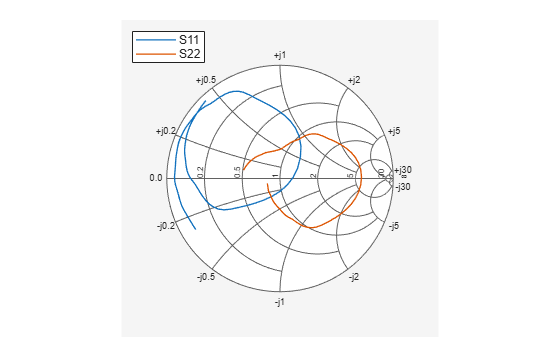Documentation

This is machine translation

Mouseover text to see original. Click the button below to return to the English version of the page.

RF Circuit Objects

This example shows how to create and use RF Toolbox™ circuit objects. In this example, you create three circuit (`rfckt`) objects: two transmission lines and an amplifier. You visualize the amplifier data using RF Toolbox™ functions and retrieve frequency data that was read from a file into the amplifier `rfckt` object. Then you analyze the amplifier over a different frequency range and visualize the results. Next, you cascade the three circuits to create a cascaded `rfckt` object. Then you analyze the cascaded network and visualize its S-parameters over the original frequency range of the amplifier. Finally, you plot the `S11`, `S22`, and `S21` parameters and noise figure of the cascaded network.

Create `rfckt` Objects

Create three circuit objects: two transmission lines, and an amplifier using data from `default.amp` data file.

```FirstCkt = rfckt.txline; SecondCkt = rfckt.amplifier('IntpType','cubic'); read(SecondCkt,'default.amp'); ThirdCkt = rfckt.txline('LineLength',0.025,'PV',2.0e8); ```

View Properties of `rfckt` Objects

You can use the `get` function to view an object's properties. For example,

```PropertiesOfFirstCkt = get(FirstCkt) ```
```PropertiesOfFirstCkt = struct with fields: LineLength: 0.0100 StubMode: 'NotAStub' Termination: 'NotApplicable' Freq: 1.0000e+09 Z0: 50.0000 + 0.0000i PV: 299792458 Loss: 0 IntpType: 'Linear' nPort: 2 AnalyzedResult: [] Name: 'Transmission Line' ```
```PropertiesOfSecondCkt = get(SecondCkt) ```
```PropertiesOfSecondCkt = struct with fields: NoiseData: [1x1 rfdata.noise] NonlinearData: [1x1 rfdata.power] IntpType: 'Cubic' NetworkData: [1x1 rfdata.network] nPort: 2 AnalyzedResult: [1x1 rfdata.data] Name: 'Amplifier' ```
```PropertiesOfThirdCkt = get(ThirdCkt) ```
```PropertiesOfThirdCkt = struct with fields: LineLength: 0.0250 StubMode: 'NotAStub' Termination: 'NotApplicable' Freq: 1.0000e+09 Z0: 50.0000 + 0.0000i PV: 200000000 Loss: 0 IntpType: 'Linear' nPort: 2 AnalyzedResult: [] Name: 'Transmission Line' ```

List Methods of `rfckt` Objects

You can use the `methods` function to list an object's methods. For example,

```MethodsOfThirdCkt = methods(ThirdCkt); ```

Change Properties of `rfckt` Objects

Use the `get` function or Dot Notation to get the line length of the first transmission line.

```DefaultLength = FirstCkt.LineLength; ```

Use the `set` function or Dot Notation to change the line length of the first transmission line.

```FirstCkt.LineLength = .001; NewLength = FirstCkt.LineLength; ```

Plot the Amplifier S11 and S22 Parameters

Use the `smithplot` method of circuit object to plot the original `S11` and `S22` parameters of the amplifier (`SecondCkt`) on a Z Smith chart. The original frequencies of the amplifier's S-parameters range from 1.0 GHz to 2.9 GHz.

```figure smithplot(SecondCkt,[1 1;2 2]); ```Plot the Amplifier Pin-Pout Data

Use the `plot` method of circuit object to plot the amplifier (`SecondCkt`) Pin-Pout data, in dBm, at 2.1 GHz on an X-Y plane.

```plot(SecondCkt,'Pout','dBm') legend('show','Location','northwest'); ```Get the Original Frequency Data and the Result of the Analyzing the Amplifier over the Original Frequencies

When the RF Toolbox reads data from default.amp into an amplifier object (`SecondCkt`), it also analyzes the amplifier over the frequencies of network parameters in default.amp file and store the result at the property `AnalyzedResult`. Here are the original amplifier frequency and analyzed result over it.

```f = SecondCkt.AnalyzedResult.Freq; data = SecondCkt.AnalyzedResult ```
```data = rfdata.data with properties: Freq: [191x1 double] S_Parameters: [2x2x191 double] GroupDelay: [191x1 double] NF: [191x1 double] OIP3: [191x1 double] Z0: 50.0000 + 0.0000i ZS: 50.0000 + 0.0000i ZL: 50.0000 + 0.0000i IntpType: 'Cubic' Name: 'Data object' ```

Analyze the Amplifier over a New Frequency Range and Plot Its New S11 and S22

To visualize the S-parameters of a circuit over a different frequency range, you must first analyze it over that frequency range.

```analyze(SecondCkt,1.85e9:1e7:2.55e9); smithplot(SecondCkt,[1 1;2 2],'GridType','ZY'); ```Create and Analyze a Cascaded `rfckt` Object

Cascade three circuit objects to create a cascaded circuit object, and then analyze it at the original amplifier frequencies which range from 1.0 GHz to 2.9 GHz.

```CascadedCkt = rfckt.cascade('Ckts',{FirstCkt,SecondCkt,ThirdCkt}); analyze(CascadedCkt,f); ```Plot the S11 and S22 Parameters of the Cascaded Circuit

Use the `smithplot` method of circuit object to plot `S11` and `S22` of the cascaded circuit (`CascadedCkt`) on a Z Smith chart.

```smithplot(CascadedCkt,[1 1;2 2],'GridType','Z'); ```Plot the S21 Parameters of the Cascaded Circuit

Use the `plot` method of circuit object to plot `S21` of the cascaded circuit (`CascadedCkt`) on an X-Y plane.

```plot(CascadedCkt,'S21','dB') legend show ```Plot the Budget S21 Parameters and Noise Figure of the Cascaded Circuit

Use the `plot` method of circuit object to plot the budget `S21` parameters and noise figure of the cascaded circuit (`CascadedCkt`) on an X-Y plane.

```plot(CascadedCkt,'budget','S21','NF') legend show ```